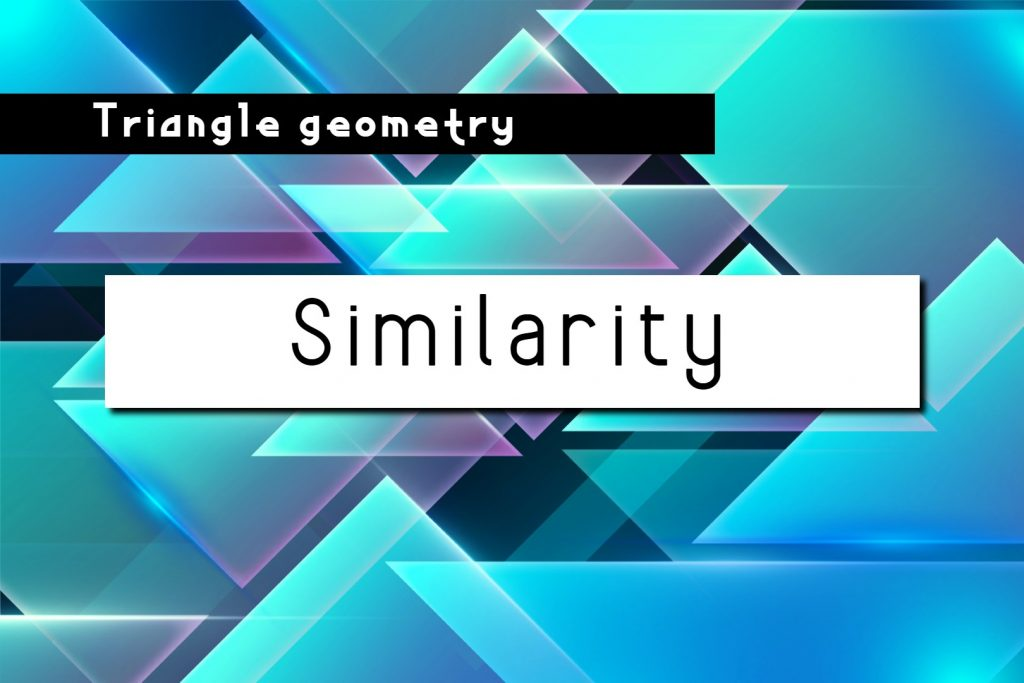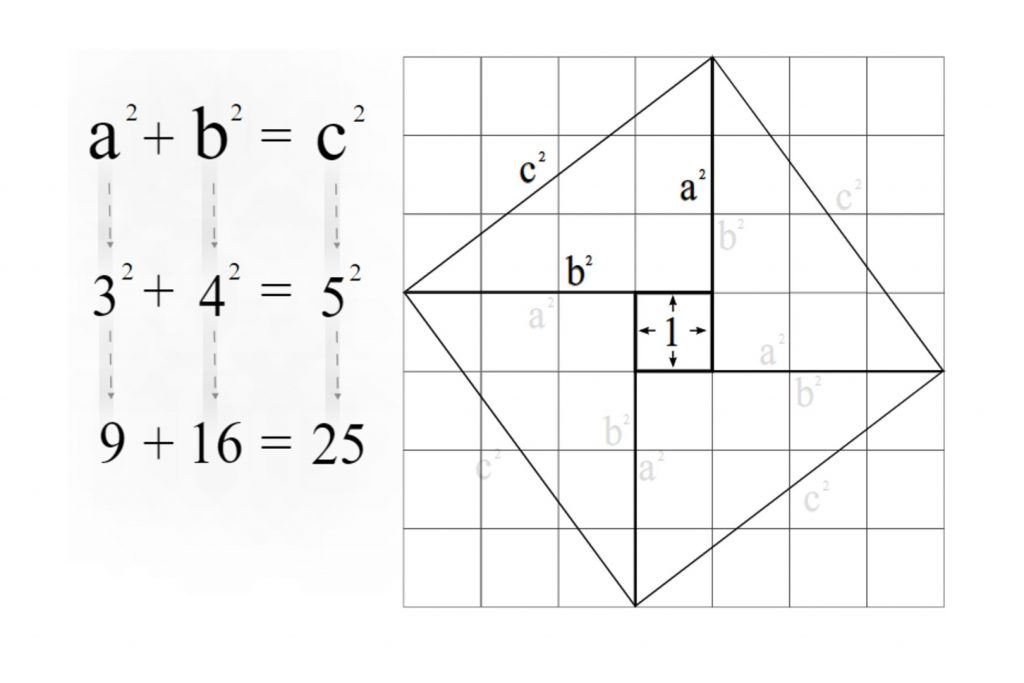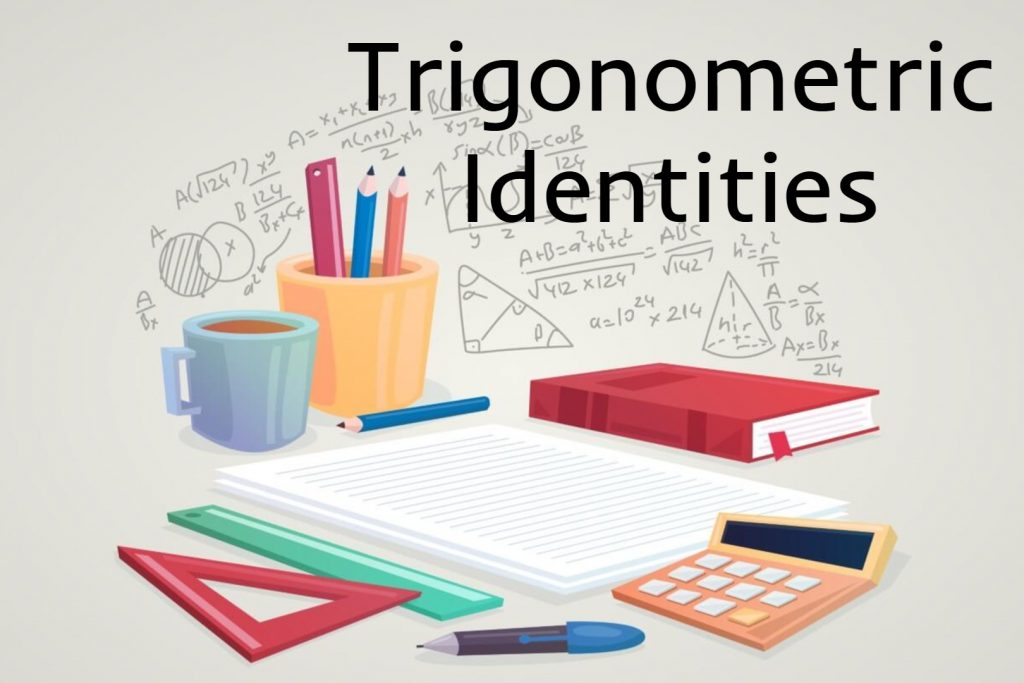# Geometry

Geometry## Similarity

Similarity,In what follows, we’ll see that many—if not most—of our results shall rely on the proportionality of sides in similar triangles. A convenient statement is as follows. Similarity, Given the similar triangles ΔABC ∼ ΔA’BC’,We have that, Conversely, if then triangles ΔABC ∼ ΔA’BC’ are similar. Proof Note first that ΔAA’C’ and ΔCA’C’ clearly have …## The Pythagorean Theorem

Right-angled triangle and the Pythagorean theorem In this article lets try to develop the Pythagorean theorem by means of the right angle. So first let’s get some proper ideas about the right-angled triangle. What is a right-angled triangle? If an angle of a triangle is 90o it is called a right-angled triangle or right triangle. …## Trigonometric Identities

A Trigonometric identity or trig identity is an identity that contains the trigonometric functions sine(sin), cosine(cos), tangent(tan), cotangent(cot), secant(sec), or cosecant(csc). Trigonometric identities can use to: Simplify trigonometric expressions. Solve trigonometric equations. Prove that one trigonometric expression is equivalent to another so that we can replace the first expression by the second expression. The following …## Role of Euclidean Geometry in High-School Mathematics

If only because in one’s “further” studies of mathematics, the results (i.e., theorems) of euclidean geometry appear only infrequently, this subject has come under frequent scrutiny, especially over the past 50 years, and at various stages, its very inclusion during a high-school mathematics curriculum has even been challenged. However, as long as we still think …